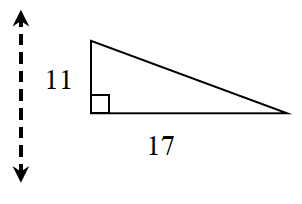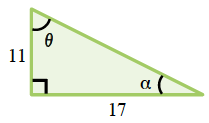### Home > CCG > Chapter Ch8 > Lesson 8.1.5 > Problem8-54

8-54.Examine the triangle and line of reflection at right.

1. On your paper, trace the triangle and the line of reflection. Then draw the image of the triangle after it is reflected across the line. Verify your reflection is correct by folding your paper along the line of reflection.

Review reflection using Math Notes box in Lesson 1.2.1.

2. Find the perimeter of the image. How does it compare with the perimeter of the original figure?

The perimeter of the reflection is the same as the perimeter of the original because all transformations are congruent to the original (isometries).

3. Find the measure of both acute angles in the original triangle.

You have learned many different trigonometry tools. Which is appropriate to the situation?

$\theta =\tan^{-1}\left (\frac{17}{11} \right ) \approx 57.1º$

Because of the Triangle Angle Sum Theorem, $\alpha$ must be $\approx 32.9º$.

Label the acute angles, like in the diagram below.$\tan(\theta) = \frac{17}{11}$